# 18.           Test

Once the card realise, we were able to make the first test.

## 18.1.                Power supply

This was the first part tested because the other component will be affected by its quality. We first isolated the supply circuit. Then we verified we obtain the two desired voltages according to different load resistances.

### 18.1.1.  5V output

The 5V voltage is stable but inferior to 5V (4,95V) if the power supply is inferior to 24V and the load is weak. The regulator 24V that consumes 2V insures 24V only if we have 26V in input. We note also the supply unit provides sufficient current to supply all the components.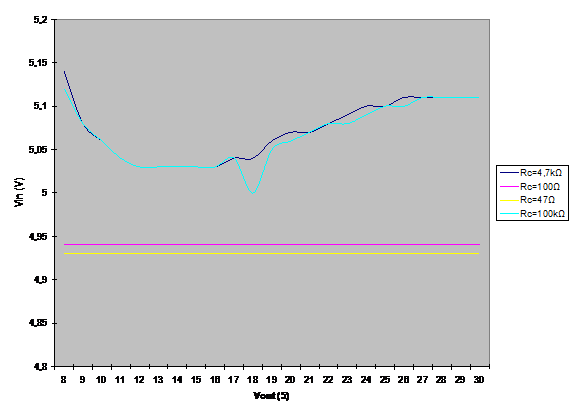Figure 38: 5v output Vs input voltage

### 18.1.2.  20V output

We observed an oscillation at the output of the comparator if we have an input voltage around 18 V (transition voltage). This phenomenon is due to a delay to charge and discharge the capacitor and leads to a loss of voltage. In the maximum oscillation, we have only 15,5V on this output. Nevertheless, this oscillation disappeared if load resistor is below 10kΩ (which is our case). Moreover, we do not use a power supply around 18V. The MMR provides 24V and the SMR 12V.

A solution to solve this problem could be to implement a hysteresis comparator instead of a simple comparator.Figure 39: 20V output Vs input voltage

As we observe on the Figure 39, the supply unit provide a voltage between 16 V and 24 V all over the input range. The flip coil driver will efficiently deliver a pulse greater than 4A.

### 18.1.3.  Efficiency and power consumption

The last test of this part has been to evaluate the consumption and the efficiency of the system. We can note that the stronger the load resistance is the lower the efficiency will be. The efficiency of the supply unit is evaluated at 55% on the SMR, and 40% on the MMR.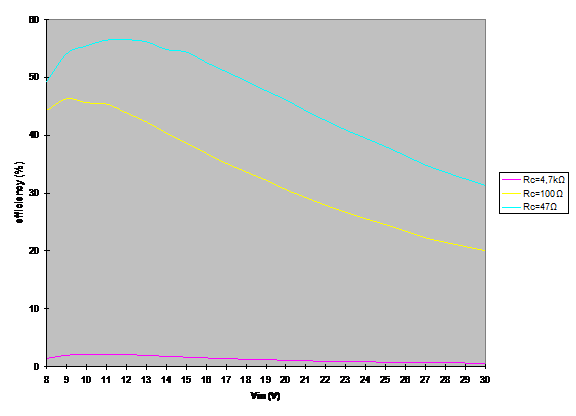Figure 40: Efficiency Vs input voltageFigure 41: Power noise

We remark that all the reference voltage are stable and oscillate just between two LSB ( which is equal to 4 mV on the microcontroller).

## 18.2.                Amplifier

Finally we had to remove this component on the circuit with the configuration showed on the Figure 8: Amplification schematic 2. We obtained unexpected output: above a certain voltage, the amplification is inverted. Unfortunately, it has also been an error on the command of the package and the amplifier we received were limited in output between V- + 0,65 and V+ - 0,65.

These two errors lead us to reconsider the gain of the magneto sensors.We do not succeed to adjust the voltage to avoid the saturation of this amplifier. Consequently, the function to process the output of the accelerometer cannot be realised by a hardware system.

The previous problem with the instrument amplifier has not occurred for the magneto sensor. We can use the amplifier INA2126 but only in the configuration proposed in data sheet (see Error! Reference source not found.). It is possible to use this amplifier for this sensor because we need a gain greater than 5.

## 18.3.                Accelerometer

As specified in the datasheet, the two output are close to 1.25V (1.31V for the x-axis and 1,29V for the y-axis) when the robot does not move on a flat area. These two values are not stable over a long period (variation inferior to 10 mV that corresponds to an acceleration of 20mg). This error could be compensating with the calibration of the sensors. The operating error due to the noise is inferior to 0.1°. We remark on the following figure that the accelerometers are not too noisy. Nevertheless, we have implemented a numeric filter to improve the quality of the measurement.Figure 42: accelerometer noise at 0g

The following figure shows the difference between the inclination read from the accelerometer and a test board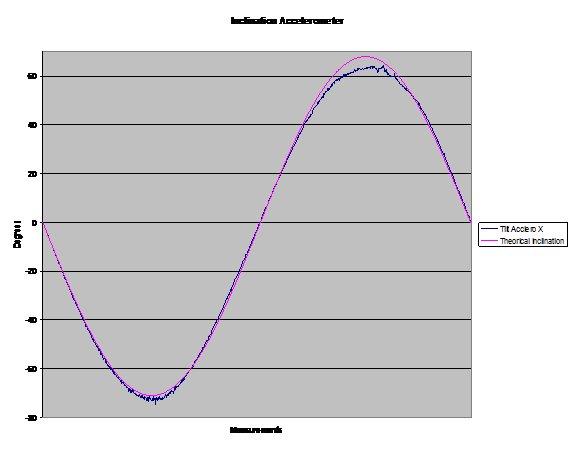## 18.4.                Magneto sensorsFigure 43: Magneto white noise

The magnetos outputs, contrary to the two previous sensors, are more stable but the mean value drift over the time if no pulse are generated (which is the case on the Figure 43). To reduce the influence of this problem and to avoid an error in when operating, a pulse is done every minute and the mean value is recalculated during the run time.

The main purpose of the system is to calculate the azimuth. To test the accuracy of the magneto, we compared the azimuth calculated from the magneto sensors with a protractor:

The figure Figure 44 demonstrates the influence of the tilt angle on the azimuth before and after the correction. To elaborate this test, the smart compass card has been inclined and the azimuth has then been calculated.Figure 44: Azimuths / inclination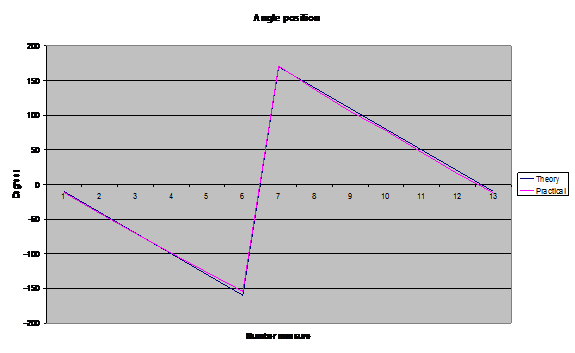Figure 45: Magneto Vs protractor

This test have been realised without tilt compensation and without inclination. We obtain these result by measuring the angle with a protractor and calculate the azimuth from the Hx and Hy field read on the magneto sensor. We have a relative difference between 0.5 and 5º due to reading error on the protractor (resolution of 1°) that may have occurs.

We also quickly tested the dependence of the azimuth versus the inclination angle. We have not precisely measure the inclination of the tilt. However, we observe that, once compensated, the azimuth is close to 0 if the inclination is below 5 º and does not exceed 4 º if the inclination is below 30 º.

We observed, during the test a change in sensitivity to an applied field in the transverse or cross-axis direction. When the magneto sensors are mounted orthogonally to each other in such a way that the x sensor is mounted in the x-y plane, y sensor in the y-x plane and z sensor in the z-y plane, then the outputs of different sensors are coupled to each other. The output of the x sensor depends on the y field. Assume that it doesnt depend to z field since that direction is perpendicular to the field plane, which is very insensitive. Likewise, the output of y sensor depends on the x field, and the output of the z sensor depends on the y field. A part of the error induced by this effect is already compensated by means of the using of the Set/Reset coil circuit.

To compensate this error, the application note could be helpful.

## 18.5.                Gyroscope

We have noticed that the output is not fixed to 2,5V as explained in the datasheet but was centred on 2,33V. As we tested it before to make the PCB, we decided to remove the instrumentation amplifier at the output of the gyro. We no longer need to centre the output to reference voltage of the ADC to avoid that this last one to saturate.Figure 46: Gyro drift

In a first time, we measured the variation of the angular rate between the gyro and the magneto on a stable state, with of a period of 1 second during 1 hour. The results of this test are exposed on the file. We remark that the angular rate from the gyroscope is more accurate than from the magneto. However, the rate from the gyro drifts over the time. Once integrated, we have a variation of 4° after one and half minute of operation and 100° after an hour. So that we can conclude that, the gyro is more accurate than the magneto at one precise moment and after a calibration.

In these conditions, if we want to stay as accurate as possible, when the magneto is disable (disturbed by interference), we can not switch on the gyro more than one minute or two. In this purpose, a time counter has been added to the method that calculate the azimuthFigure 47 Magneto / gyro

The Figure 47 shows the results of the difference between the gyroscope and the azimuth calculated from the magnetic field when the card is placed on a robot over a rotation of 360°. These values are extracted from the Figure 48 where the robot measurement starts when the robot is stopped. Then this latter turns 720° before to stop again. The yellow curve is the azimuth calculated from the magnetic field shown on the Figure 49. Once recentered on 0 (Hx-87 Hy+240 compensated). We obtain the blue curves. That is close to the gyroscope value. This compensation shows the effect of the hard Iron effect due to the SMR to the smart compass.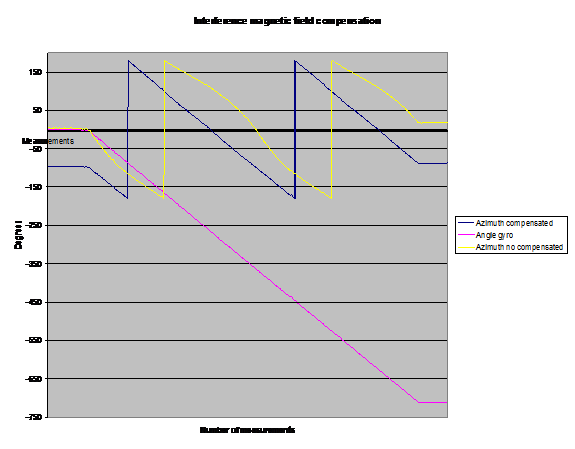Figure 48: test on 720°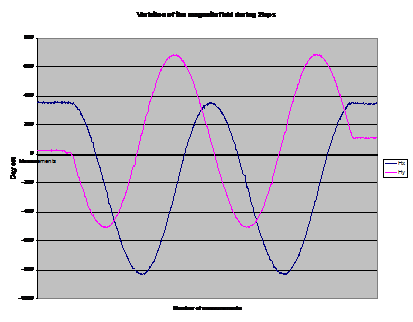Figure 49: Hx and Hy

## 18.6.                The clock

The most important problem on the first card has been the operating frequency of the microcontroller. We have not succeeded to synchronise the MCU with the external clock at 14 MHz. We have tested different quartz and different capacitor but it has never worked in this configuration. So we have worked with the internal clock of the microcontroller at 8 MHz.

On the second card, we have increased the value of the decoupling capacitor between the microcontroller and the pulse circuit. The circuit has worked with the external clock during one week, but after this period, the microcontroller has suddenly consumed unusual current and has not worked with the external clock since. We use now the internal clock at 4 MHz. To replace the ATMEGA32 would probably allow to reusing the external clock, but with a risk to have again this problem because we havent identify its source but we thought a short circuit between the metallic envelop and the 5 volt plane main be in cause.

This problem has been solved by changing the ATmega32. The controller now run at 16Mh

## 18.7.                The magnetic field compensation

We first simulate the circuit fallowing circuit: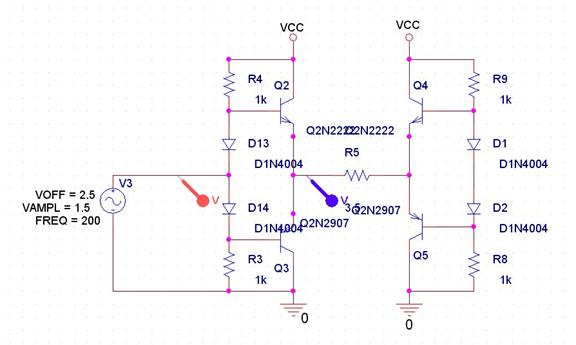Figure 50: Amplifier schematicFigure 51: Compensation simulation

This simulation encourages us to implement the circuit on the first card. However, when we tested it with the digital potentiometer, we realised that the impedance was not adapted between the voltage divider and the amplifier. Therefore, we are far from the simulated characteristic.

On the second card, where the schematic has been simplified, we then have the expected drive voltage. We obtained the following results:Figure 52: Magnetic vector measurement after a compensation on the X-axis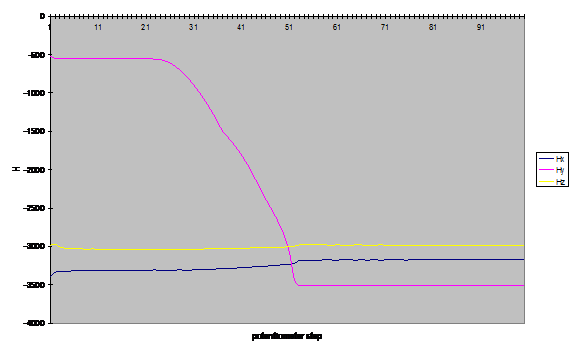Figure 53: Magnetic vector measurement after a compensation on the Y-axis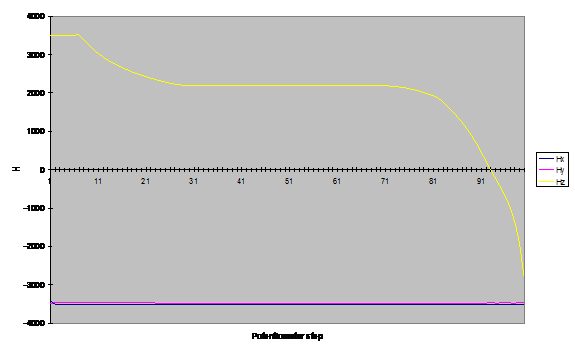Figure 54: Magnetic vector measurement after a compensation on the Z-axis

We discovered a second problem on the compensation circuit: The axes X interfered with the Y axe. We do not discover yet the origin of that error. It may be an error on the magneto sensor or the result of a wrong measurement.

The stage on the compensation is due to the cross distortion or from the saturation of the amplifier: The field compensated can have a magnitude of  0,5 Gauss.

In conclusion to this test, the compensation work well for the Y and Z axes (after a translation from a table). The X axis dependence has to be fixed.

## 18.8.                The temperature

We remark on these figures that the outputs voltage of the temperature increase during the running time. If we convert these voltages to temperature, we can see these variations correspond only to a maximum of 2° C. Consequently, this parameter does not influence the gyroscope and the accelerometer in normal use. We have calculated it should have a minimum variation of 5° to influence the sensitivity of these two components.Figure 55: Temperature variation

## 18.9.                Filter

To decrease the influence of the noise we have tested two filters: the first one is a moving average filter. It is a simple filter modelize by the following equation:

Averagen = (Averagen-1 + Outputn) / 2

Equation 11: Average filter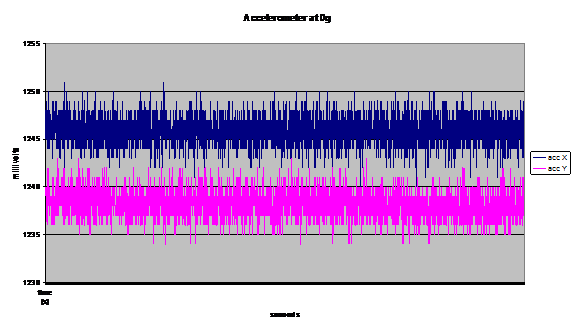Figure 56: Accelerometer not filtered

If we apply this filter on the accelerometer result (Figure 56), then we obtain the following result (Figure 57):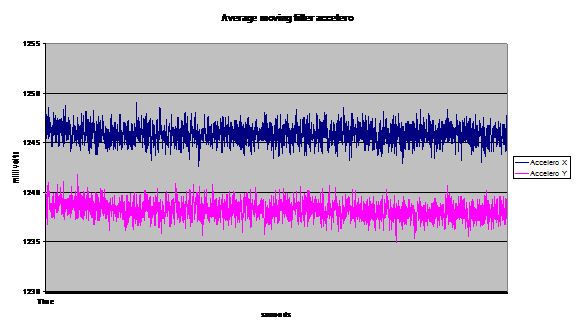Figure 57Accelerometer with an average filter

We can see that this kind of filter decrease a great part of the noise. We have then tested a numeric low pass filter of the first order:

Outputn = A * Inputn + (1 - A) * Outputn-1

A = (1 / SR) / (1 / SR + 1 / Fc)

SR: sampling rate

Fc: 3db breakpoint

Equation 12: numerical low pass filter

As the acquisition of each data has been done every 1s, we have decided to test the filter at 0.01 Hz.. From this filter we get the Figure 46: Gyro drift.

As expected, the noise decreased and a delay appears.

Note: We have implemented this numeric filter in every module. Each breakpoint can be set-up in the constructer of the module (ex: CAccelero())